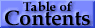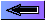3.   Atomic States, Shells, and Configurations

A one-electron atomic state is defined by the quantum numbers nlmlms or nljmj, with n and l representing the principal quantum number and the orbital angular momentum quantum number, respectively. The allowed values of n are the positive integers, and l =  0, 1, ..., n - 1. The quantum number j represents the angular momentum obtained by coupling the orbital and spin angular momenta of an electron, i.e., j = l + s, so that j = l ± 1/2. The magnetic quantum numbers ml, ms, and mj represent the projections of the corresponding angular momenta along a particular direction; thus, for example, ml = -l, -l + 1 ... l and ms = ± 1/2.

The central field approximation for a many-electron atom leads to wave functions expressed in terms of products of such one-electron states [2,3]. Those electrons having the same principal quantum number n belong to the shell for that number. Electrons having both the same n value and l value belong to a subshell, all electrons in a particular subshell being equivalent. The notation for a configuration of N equivalent electrons is nl N, the superscript usually being omitted for N = 1. A configuration of several subshells is written as nl Nnl M ... . The numerical values of l are replaced by letters in writing a configuration, according to the code s, p, d for l = 0, 1, 2 and f, g, h ... for l = 3, 4, 5 ..., the letter j being omitted.

The Pauli exclusion principle prohibits atomic states having two electrons with all four quantum numbers the same. Thus the maximum number of equivalent electrons is 2(2l + 1). A subshell having this number of electrons is full, complete, or closed, and a subshell having a smaller number of electrons is unfilled, incomplete, or open. The 3p6 configuration thus represents a full subshell and 3s2 3p6 3d10 represents a full shell for n = 3.

The parity of a configuration is even or odd according to whether Σili is even or odd, the sum being taken over all electrons (in practice only those in open subshells need be considered).

4.   Hydrogen and Hydrogen-like Ions

The quantum numbers n, l, and j are appropriate . A particular level is denoted either by nlj or by nl 2LJ with L = l and J = j. The latter notation is somewhat redundant for one-electron spectra, but is useful for consistency with more complex structures. The L values are written with the same letter code used for l values, but with roman capital letters. The multiplicity of the L term is equal to 2S + 1 = 2s + 1 = 2. Written as a superscript, this number expresses the doublet character of the structure: each term for L ≥ 1 has two levels, with J = L ± 1/2, respectively.

The Coulomb interaction between the nucleus and the single electron is dominant, so that the largest energy separations are associated with levels having different n. The hyperfine splitting of the 1H 1s ground level [1420.405 751 766 7(10) MHz] results from the interaction of the proton and electron magnetic moments and gives rise to the famous 21 cm line. The separations of the 2n - 1 excited levels having the same n are largely determined by relativistic contributions, including the spin-orbit interaction, with the result that each of the n - 1 pairs of levels having the same j value is almost degenerate; the separation of the two levels in each pair is mainly due to relatively small Lamb shifts.

5.   Alkalis and Alkali-like Spectra

In the central field approximation there exists no angular-momentum coupling between a closed subshell and an electron outside the subshell, since the net spin and orbital angular momenta of the subshell are both zero. The nlj quantum numbers are, then, again appropriate for a single electron outside closed subshells. However, the electrostatic interactions of this electron with the core electrons and with the nucleus yield a strong l-dependence of the energy levels . The differing extent of "core penetration"" for ns and np electrons can in some cases, for example, give an energy difference comparable to or exceeding the difference between the np and (n + 1)p levels. The spin-orbit fine-structure separation between the nl  (l > 0) levels having j = l - 1/2 and l + 1/2, respectively, is relatively small.